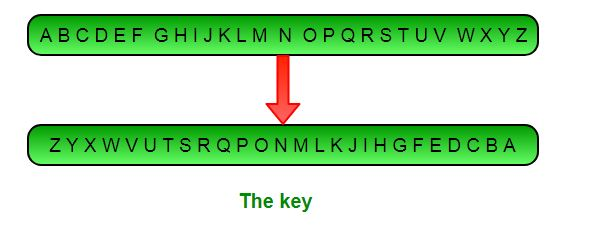# Implementing Atbash Cipher

• Difficulty Level : Easy
• Last Updated : 12 Jul, 2022

Definition: Atbash cipher is a substitution cipher with just one specific key where all the letters are reversed that is A to Z and Z to A. It was originally used to encode the Hebrew alphabets but it can be modified to encode any alphabet.

Relationship to Affine: Atbash cipher can be thought of as a special case of Affine cipher with both the keys being 25, i.e, a = 25 & b = 25Atbash Key

Algorithm: The following key is used in the Atbash algorithm

```ABCDEFGHIJKLMNOPQRSTUVWXYZ
ZYXWVUTSRQPONMLKJIHGFEDCBA```

Encryption

To encipher a message, find the letter you wish to encipher in the top row, then replace it with the letter in the bottom row. In the example below, we encipher the message ‘GEEKS FOR GEEKS’. The first letter we wish to encipher is ‘G’, which is above ‘T’, so the first ciphertext letter is ‘T’. The next letter is ‘E’, which is above ‘V’, so that comes next. The whole message is enciphered as:

```GEEKS FOR GEEKS
TVVPH ULI TVVPH```

Decryption

Similarly if we want to decrypt say ‘TVVPH ULI TVVPH’ we would replace the first letter ‘T’ with ‘G’. The second letter ‘V’ with ‘E’ and so forth substituting all letters including the last one ‘H’ with ‘S’. The deciphered message will be :

```TVVPH ULI TVVPH
GEEKS FOR GEEKS```

The Approach: Here, mapping of every element is done for the key as ‘key-value’ pairs in a dictionary and then it is used as a lookup table whenever you want to encrypt a single character. Code:

Implementation:

## Python

 `# Python program to implement Atbash Cipher` `# This script uses dictionaries to lookup various alphabets``lookup_table ``=` `{``'A'` `: ``'Z'``, ``'B'` `: ``'Y'``, ``'C'` `: ``'X'``, ``'D'` `: ``'W'``, ``'E'` `: ``'V'``,``        ``'F'` `: ``'U'``, ``'G'` `: ``'T'``, ``'H'` `: ``'S'``, ``'I'` `: ``'R'``, ``'J'` `: ``'Q'``,``        ``'K'` `: ``'P'``, ``'L'` `: ``'O'``, ``'M'` `: ``'N'``, ``'N'` `: ``'M'``, ``'O'` `: ``'L'``,``        ``'P'` `: ``'K'``, ``'Q'` `: ``'J'``, ``'R'` `: ``'I'``, ``'S'` `: ``'H'``, ``'T'` `: ``'G'``,``        ``'U'` `: ``'F'``, ``'V'` `: ``'E'``, ``'W'` `: ``'D'``, ``'X'` `: ``'C'``, ``'Y'` `: ``'B'``, ``'Z'` `: ``'A'``}` `def` `atbash(message):``    ``cipher ``=` `''``    ``for` `letter ``in` `message:``        ``# checks for space``        ``if``(letter !``=` `' '``):``            ``#adds the corresponding letter from the lookup_table``            ``cipher ``+``=` `lookup_table[letter]``        ``else``:``            ``# adds space``            ``cipher ``+``=` `' '` `    ``return` `cipher` `# Driver function to run the program``def` `main():``    ``#encrypt the given message``    ``message ``=` `'GEEKS FOR GEEKS'``    ``print``(atbash(message.upper()))``    ` `    ``#decrypt the given message``    ``message ``=` `'TVVPH ULI TVVPH'``    ``print``(atbash(message.upper()))`  `# Executes the main function``if` `__name__ ``=``=` `'__main__'``:``    ``main()`

Output

```TVVPH ULI TVVPH
GEEKS FOR GEEKS```

Advantages : Since its just an affine cipher with both a = 25 = b we don’t need to write separate functions for encryption and decryption. We can re-use the same function for both purposes.

Analysis : It has one constant key it is the easiest cipher to break and provides almost no security. Anyone can assume that it is atbash and decrypt the message by reversing the letters.

This article is contributed by Palash Nigam . If you like GeeksforGeeks and would like to contribute, you can also write an article using write.geeksforgeeks.org or mail your article to review-team@geeksforgeeks.org. See your article appearing on the GeeksforGeeks main page and help other Geeks.

My Personal Notes arrow_drop_up